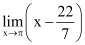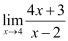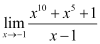# Limits and Derivatives Chapter 13 Class 11 Maths NCERT Textbook With Solutions PDF

NCERT Solutions for Class 11 Maths Chapter 13‘ PDF Quick download link is given at the bottom of this article. You can see the PDF demo, size of the PDF, page numbers, and direct download Free PDF of ‘Ncert Class 11 Maths Chapter 13 Exercise Solution’ using the download button.

### Chapter 13: Limits and Derivatives

#### 13.1 Introduction

This chapter is an introduction to Calculus. Calculus is a branch of mathematics which mainly deals with the study of change in the value of a function as the points in the domain change.

First, we give an intuitive idea of derivatives (without actually defining them).

Then we give a naive definition of a limit and study some algebra of limits. Then we come back to a definition of derivative and study some algebra of derivatives. We also obtain derivatives of certain
standard functions.

#### 13.2 Intuitive Idea of Derivatives

Physical experiments have confirmed that the body dropped from a tall cliff covers a distance of 4.9t 2 meters in t seconds, i.e., distance s in meters covered by the body as a function of time t in seconds is given by s = 4.9t2.

The adjoining Table 13.1 gives the distance travelled in metres at various intervals of time in seconds of a body dropped from a tall cliff. The objective is to find the velocity of the body at time t = 2 seconds from this data.

One way to approach this problem is to find the average velocity for various intervals of time ending at t = 2 seconds and hope that these throw some light on the velocity at t = 2 seconds.

Average velocity between t = t 1 and t = t2 equals distance travelled between t = t1 and t = t2 seconds divided by (t 2 – t 1). Hence the average velocity in the first two seconds

### NCERT Solutions Class 11 Maths Chapter 13 Limits and Derivatives

1. Evaluate the Given limit:

Solution:

Given

Substituting x = 3, we get

= 3 + 3

= 6

2. Evaluate the Given limit:

Solution:

Given limit:Substituting x = π, we get

= (π – 22 / 7)

3. Evaluate the Given limit:

Solution:

Given limit:

Substituting r = 1, we get

π(1)2

= π

4. Evaluate the Given limit:

Solution:

Given limit:Substituting x = 4, we get= [4(4) + 3] / (4 – 2)

= (16 + 3) / 2

= 19 / 2

5. Evaluate the Given limit:

Solution:

Given limit:Substituting x = -1, we get

= [(-1)10 + (-1)5 + 1] / (-1 – 1)

= (1 – 1 + 1) / – 2

= – 1 / 2